# Box Plot in R Tutorial

Learn about box plots in R, including what they are, when you should use them, how to implement them, and how they differ from histograms.
Sep 2020  · 4 min read

The `boxplot()` function shows how the distribution of a numerical variable `y` differs across the unique levels of a second variable, `x`. To be effective, this second variable should not have too many unique levels (e.g., 10 or fewer is good; many more than this makes the plot difficult to interpret).

The `boxplot()` function also has a number of optional parameters, and this exercise asks you to use three of them to obtain a more informative plot:

• `varwidth` allows for variable-width Box Plot that shows the different sizes of the data subsets.
• `log` allows for log-transformed y-values.
• `las` allows for more readable axis labels.

## When you should use a Box Plot

• When you have a continuous variable, split by a categorical variable.

• When you want to compare the distributions of the continuous variable for each category.

## Histogram vs. Box Plot

• Below is the comparison of a Histogram vs. a Box Plot. The line in the middle shows the median of the distribution. That is, half the monarchs started ruling before this age, and half after this age.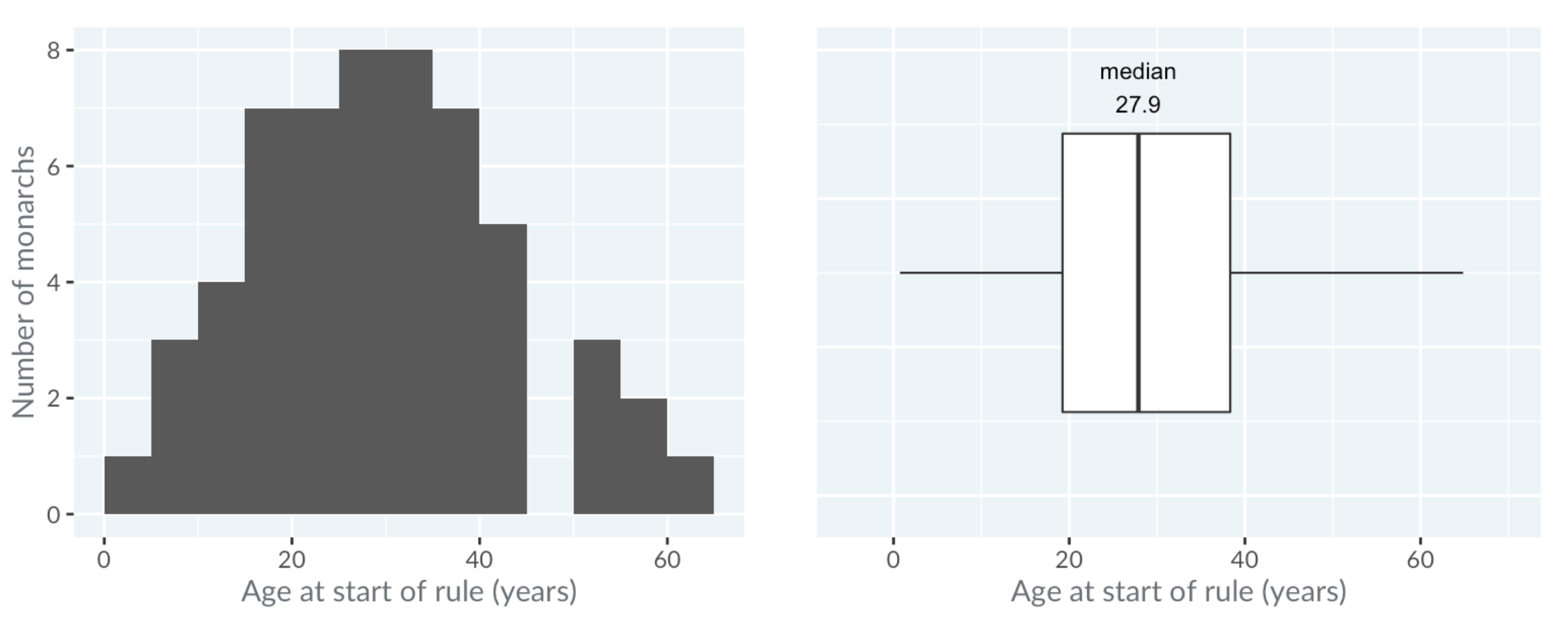• The box in the Box Plot extends from the lower quartile to the upper quartile. The lower quartile is the point where one-quarter of the values are below it. That is, one-quarter of the monarchs started ruling before this age, and three-quarters after it. Likewise, the upper quartile is the age where three quarters of the monarchs started ruling below this age. The difference between the upper quartile and the lower quartile is called the inter-quartile range.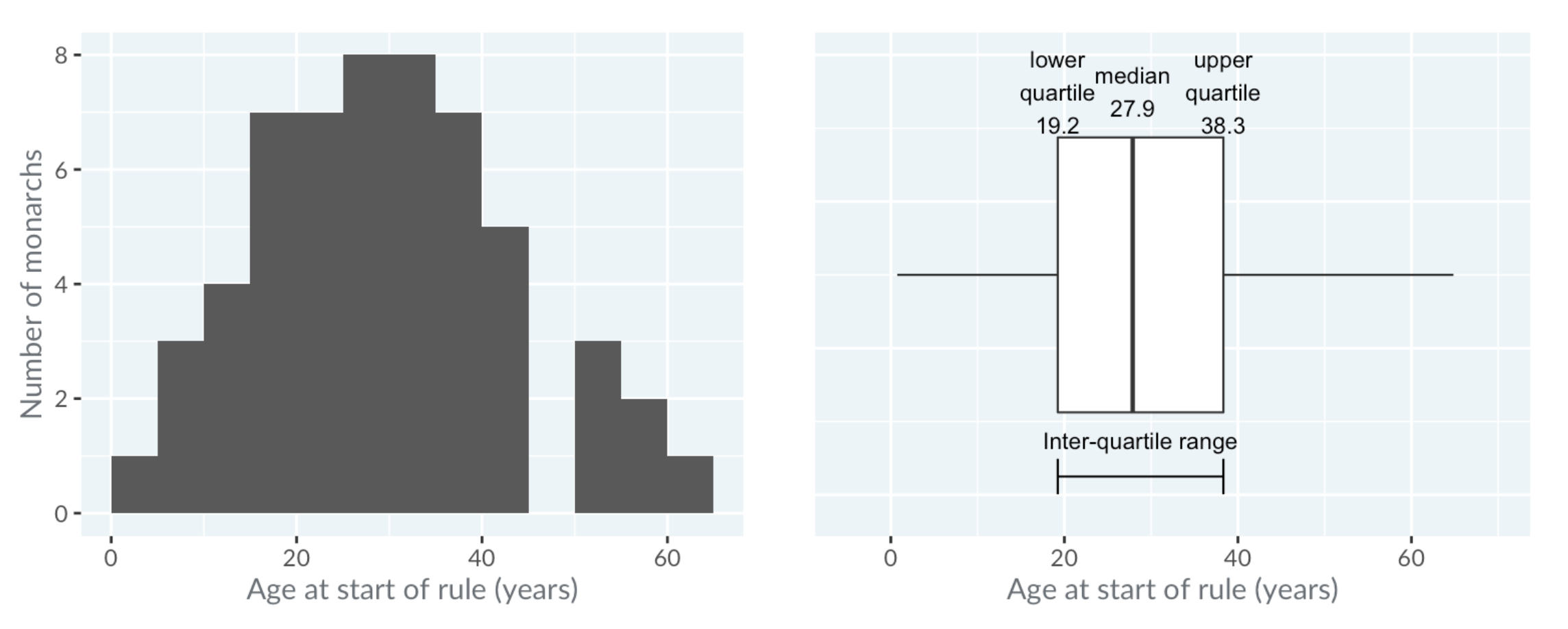The horizontal lines, known as "whiskers", have a more complicated definition. Each bar extends to one and a half times the interquartile range, but then they are limited to reaching actual data points.

The technical definition is shown in the image below, but in practice, you can think of the whiskers as extending far enough that anything outside of them is an extreme value.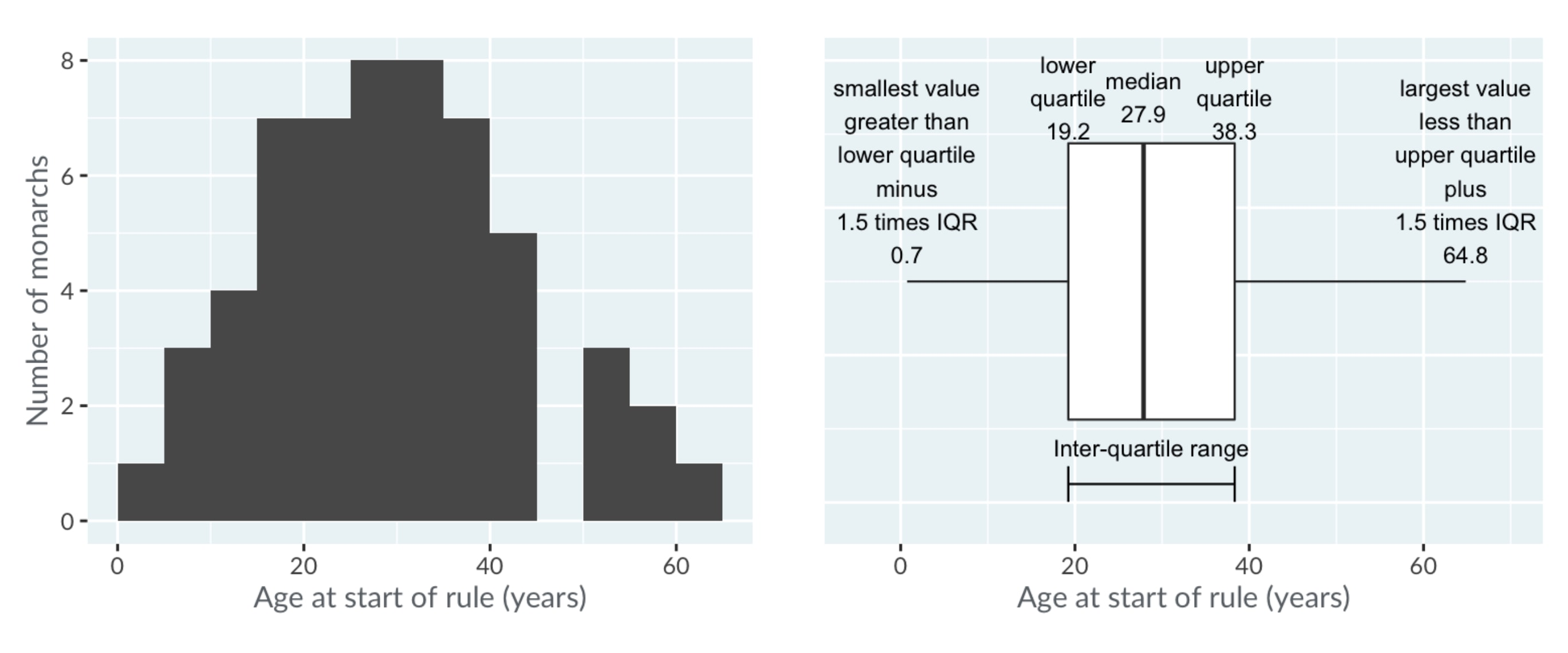As mentioned before, the power of Box Plots is that you can compare many distributions at once. Here, the royal houses are ordered from oldest at the top to newest at the bottom.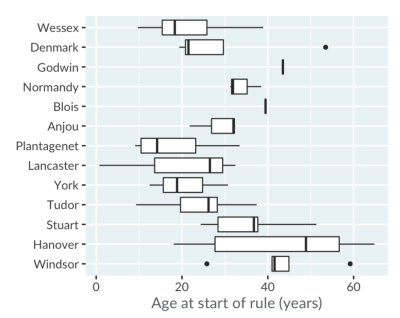A trend is visible: since the Plantagenets in the fourteenth century, the boxes gradually move right, showing that the ages when new monarchs ascend to the throne have been increasing.

Godwin and Blois appear as a single line because there was only one king from each house. The Anjou house only had three kings, and forms a box with one whisker, not two.

Notice that the Box Plots for the houses of Denmark and Windsor show some points. These are extreme values, that is, values that are outside the range of the whiskers. Windsor's left-most outlier is Elizabeth the second, who ascended at age 26.

## `boxplot()` Function

In the following example, using the formula interface, you will create a Box Plot showing the distribution of numerical `crim` values over the different distinct `rad` values from the Boston data frame. Then, use the `varwidth` parameter to obtain variable-width Box Plots, specify a log-transformed y-axis, and set the `las` parameter equal to 1 to obtain horizontal labels for both the x and y-axes.

Finally, use the `title()` function to add the title `"Crime rate vs. radial highway index"`.

``````# Create a variable-width Box Plot with log y-axis & horizontal labels
boxplot(crim ~ rad, data = Boston,
varwidth = TRUE, log = "y", las = 1)

title("Crime rate vs. radial highway index")
``````

When we run the above code, it produces the following result: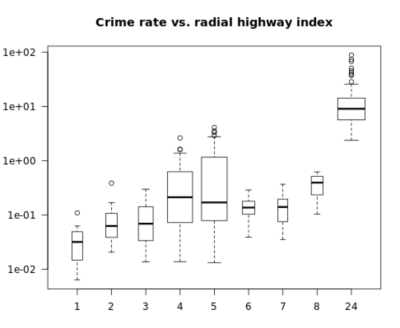This content is taken from DataCamp’s Understanding Data Visualization course by Richie Cotton and our Data Visualization in R course by Ronald Pearson.

### .css-1531qan{-webkit-text-decoration:none;text-decoration:none;color:inherit;}Introduction to R

Beginner
4 hr
2.5M learners
Master the basics of data analysis in R, including vectors, lists, and data frames, and practice R with real data sets.

### Intermediate R

Beginner
6 hr
548.7K learners
Continue your journey to becoming an R ninja by learning about conditional statements, loops, and vector functions.

### Introduction to the Tidyverse

Beginner
4 hr
274.5K learners
Get started on the path to exploring and visualizing your own data with the tidyverse, a powerful and popular collection of data science tools within R.
See More
Related

### Inside Our Favorite DataFramed Episodes of 2022

An inside look at our favorite episodes of the DataFramed podcast of 2022

2 min### [Infographic] Data Science Project Checklist

Use this checklist when planning your next data science project.

### Introduction to Probability Rules Cheat Sheet

Learn the basics of probability with our Introduction to Probability Rules Cheat Sheet. Quickly reference key concepts and formulas for finding probability, conditional probability, and more.

### Data Governance Fundamentals Cheat Sheet

Master the fundamentals of data governance with our Data Governance Fundamentals Cheat Sheet. Quickly reference key concepts, best practices, and key components of a data governance program.